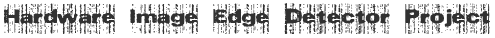Edge Detection in FPGA using Sobel Operator

### Edge Detection Theory

An edge can be defined as an abrupt change in brightness as we move from one pixel to its neighbour in an image. In digital image processing, each image is quantized into pixels. With gray-scale images, each pixel indicates the level of brightness of the image in a particular spot: 0 represents black, and with 8-bit pixels, 255 represent white. An edge is an abrupt change in the brightness (gray scale level) of the pixels. Detecting edges is an important task \in boundary detection, motion detection/estimation, texture analysis, segmentation, and object identification.
Edge information for a particular pixel is obtained by exploring the brightness of pixels in the neighborhood of that pixel. If all of the pixels in the neighborhood have almost the same brightness, then there is probably no edge at that point. However, if some of the neighbors are much brighter than the others, then there is a probably an edge at that point. Measuring the relative brightness of pixels in a neighborhood is mathematically analogous to calculating the derivative of brightness. The image illustrates an example of Hard and Soft Edges on an image. Brightness values are discrete, not continuous, so we approximate the derivative function. Different edge detection methods (Prewitt, Laplacian, Roberts, Sobel and Canny) use different discrete approximations of the derivative function.
For example consider a random discrete 9 x 9 pixel image.
Refresh the page to see randomize the pattern. Horizontal Edge Example Vertical Edge Example Diagonal Edge Example
X difference is calculated as | I(i+1,j) - I(i,j)| Y difference is calculated as | I(i,j+1) - I(i,j)| where I denotes the intensity values [0-255]

more »

### Sobel Edge Detection Operator

The Sobel Edge Detection Operator is a 3x3 Spatial mask. It is based on the differential operation [1 0 -1] and an averaging operator [1 2 1], Convolving these operators we get the 3x3 spatial mask for sobel:
-1 0 1 Horizontal Gradient Operator -2 0 2 -1 0 1
-1 -2 -1 Vertical Gradient Operator 0 0 0 1 2 1
The Spatial mask is convolved over the image to obtain the edge or high passed image. The image shows the convolution mask working over an image. The mask is convolved and the center element is replaced as the mask operates on the image. The borders of the images are usually blacked out, since they cannot be computed with a 3x3 mask, or partially computed.
Gx[i, j] = Im[i+1, j-1] + 2*Im[i+1, j] + Im(i+1, j+1) – { Im[i-1, j-1] + 2*Im[i-1, j] + Im(i-1, j+1) }
Gy[i, j] = Im[i-1, j+1] + 2*Im[i, j+1] + Im(i+1, j+1) – { Im[i-1, j-1] + 2*Im[i, j-1] + Im(i+1, j-1) }

more »

### Relevance of the Project

Edge detection is a very complex process affected by deterioration due to different level of noise. A number of operators are defined to solve the problem of edge detection. They are effective for certain classes of images, but not suitable for others. Edge detection is a crucial step in digital image processing. It has found application in artificial intelligence systems, forensic science and also in digital multimedia for creating image dazzling effect. Currently the image processing algorithms has been limited to software implementation which is slower due to the limited processor speed. So a dedicated hardware for edge detection has been required which was not possible until the advancements in VLSI technology.
Edge detection becomes a more complicated task when using much improved edge detection masks. Moreover the process becomes lengthier when it operates on an image of very high resolution. Most hardware implementations are faster than its corresponding software implementations. So implementing edge detection in hardware will be more efficient. Since FPGA have got the added feature of parallelism, the edge detection can be effectively implemented.
During the recent years, field programmable gate arrays (FPGA) have become the dominant form of programmable logic. In comparison to previous programmable devices like programmable array logic (PAL) and complex programmable logic devices (CPLD), FPGA can implement far larger logic functions. FPGA supports sufficient logic to implement complete systems and sub-systems. FPGA provides designers with reconfigurable logic that can be reprogrammed on application-specific basis. This drastically increases flexibility in the design process.
Any edge detection masks when operated on a group on 9 pixels, there will be 12 multiplications. So while processing an image of size n x n, there will be a total of 12 x (n-2)2 multiplications. The total number of multiplications can be reduced to 2 x (n-2)2 (ie. reduced about 6 times) by using Sobel edge detection operator because it has got mask values ‘1’ which does not require any multiplication at all. Moreover the multiplication by 2 can be implemented easily using a shift operation instead of implementing a multiplier.
The choice of Sobel edge detection operator is also motivated by the fact that they incorporate both the edge detection as well as smoothing operator so that they have good edge detection capability in noisy conditions. Sobel operator is less deteriorated in high levels of noise.

more »

### Edge Detection Hardware Architecture

The Block gives the overall I/O ports on the edge detection system using fpga.

The bus_rw controls the direction of data transfer while data_strobe and mode_strobe signals control the entire data transfer operation. The clk signal is the internal clock of the FPGA. The bus(7:0) is an 8 bit bidirectional data bus. Internally the system consists of Ram Modules wired to 32 FPGA modules. There are 3 sets of 34byte RAM array which can be serially loaded and parallely shifted. The 3 sets of RAM Array are wired to 32 Sobel Instances

more »